## ndarray中nan的替换（均值）

ndarray中存在nan，如何操作才能够将nan替换为每一列的均值？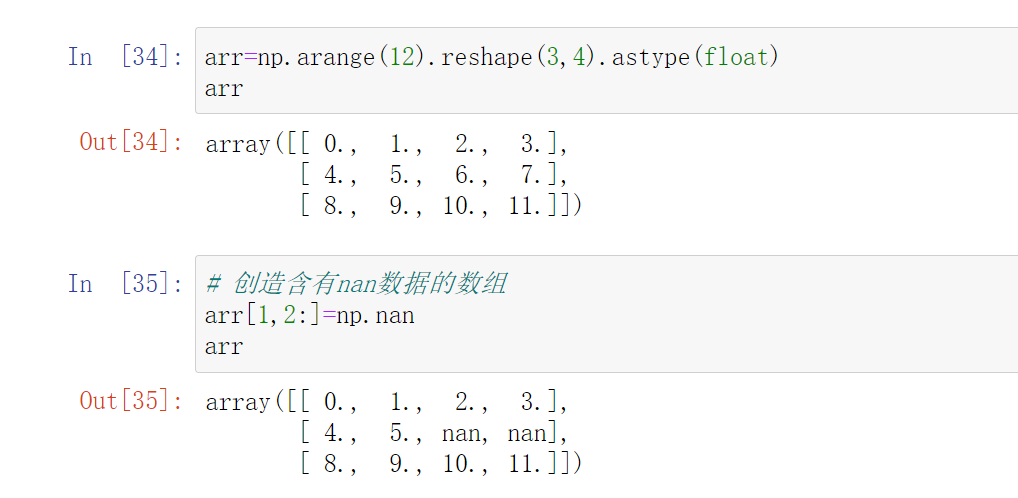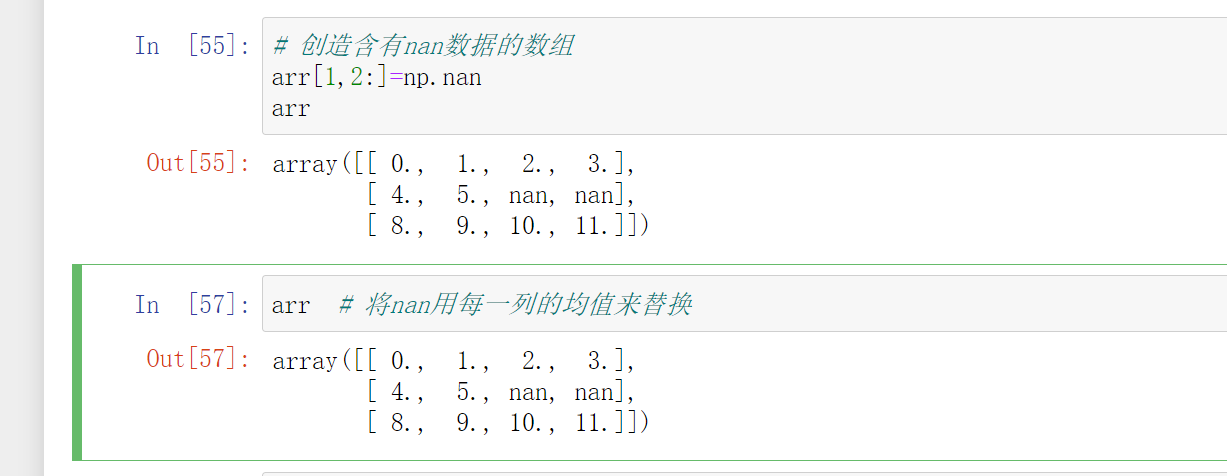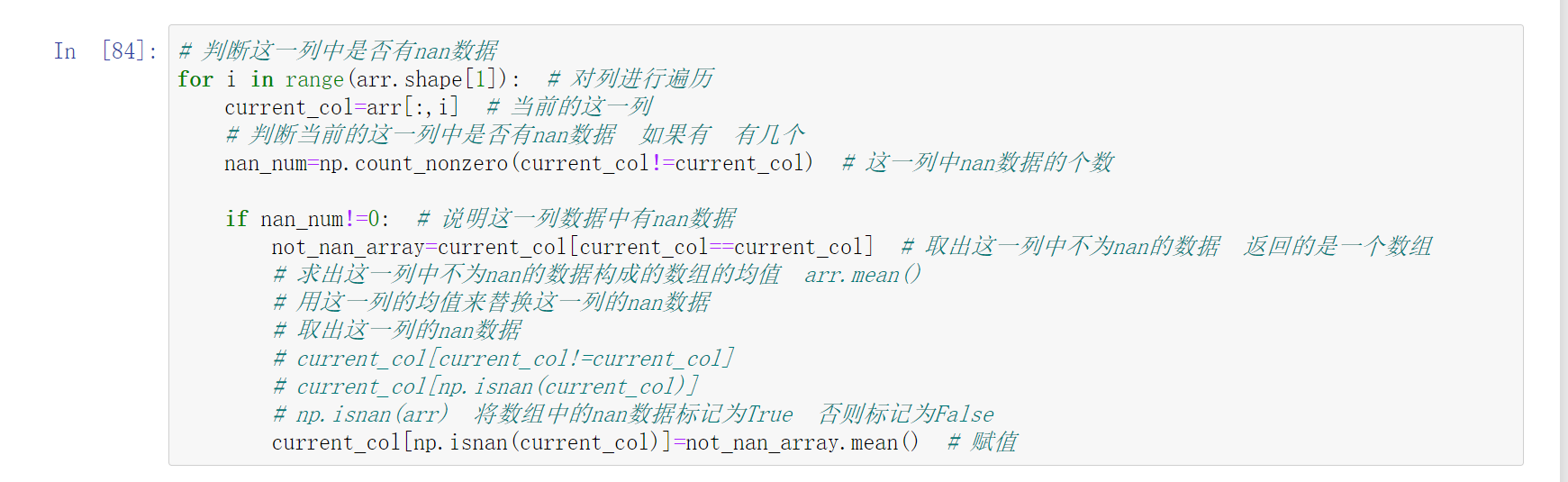``````# 判断这一列中是否有nan数据
for i in range(arr.shape):  # 对列进行遍历
current_col=arr[:,i]  # 当前的这一列
# 判断当前的这一列中是否有nan数据  如果有  有几个
nan_num=np.count_nonzero(current_col!=current_col)  # 这一列中nan数据的个数

if nan_num!=0:  # 说明这一列数据中有nan数据
not_nan_array=current_col[current_col==current_col]  # 取出这一列中不为nan的数据  返回的是一个数组
# 求出这一列中不为nan的数据构成的数组的均值  arr.mean()
# 用这一列的均值来替换这一列的nan数据
# 取出这一列的nan数据
# current_col[current_col!=current_col]
# current_col[np.isnan(current_col)]
# np.isnan(arr)  将数组中的nan数据标记为True  否则标记为False
current_col[np.isnan(current_col)]=not_nan_array.mean()  # 赋值
``````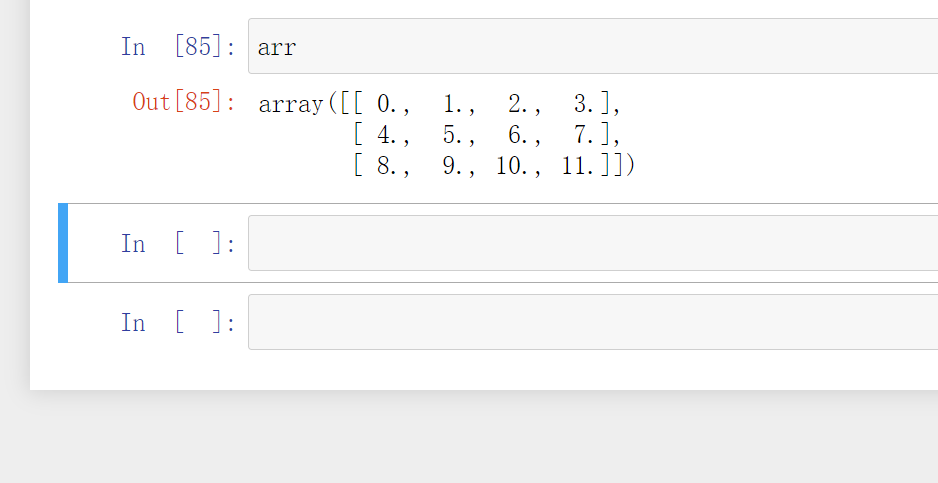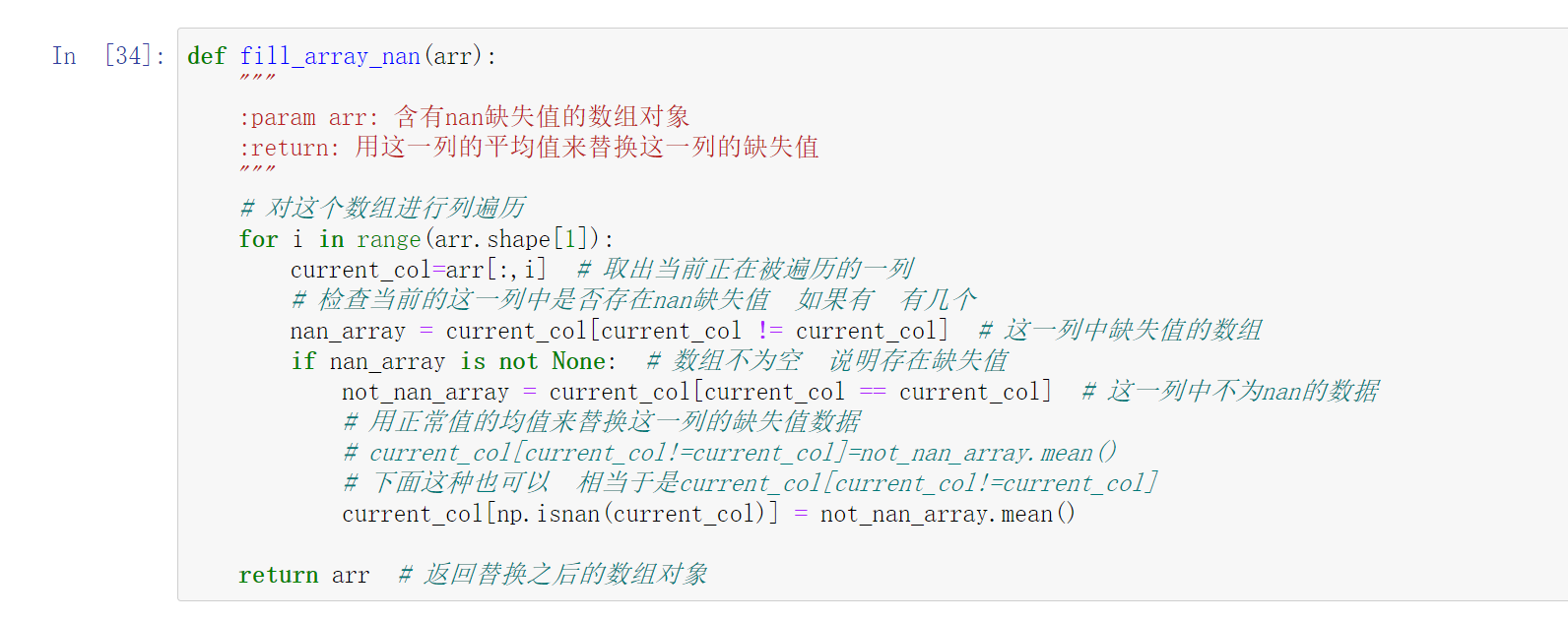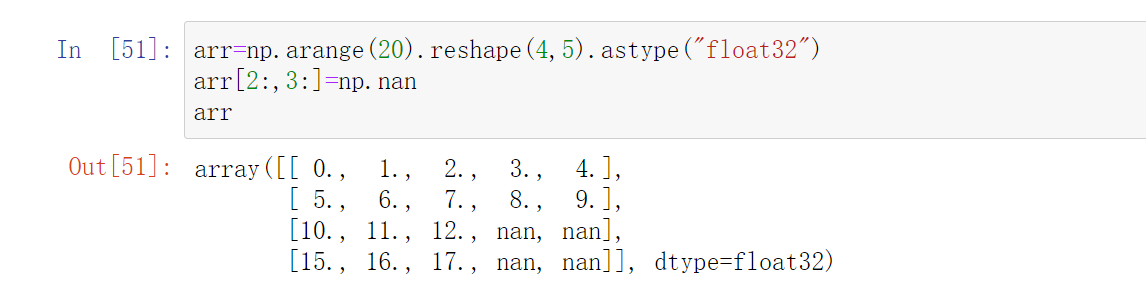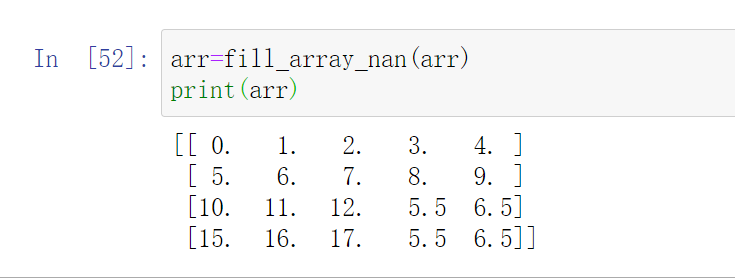``````# -*- coding: utf-8 -*-

'''
@Time    : 2020/12/05 19:49
@Author  : yuhui
@Email   : [email protected]
@FileName: numpy_2.py
@Software: PyCharm
'''

"""19【numpy中的nan和常用方法】01numpy中的nan和常用统计方法"""

import numpy as np

def fill_array_nan(arr):
"""
:param arr: 含有nan缺失值的数组对象
:return: 用这一列的平均值来替换这一列的缺失值
"""
# 对这个数组进行列遍历
for i in range(arr.shape):
current_col=arr[:,i]  # 取出当前正在被遍历的一列
# 检查当前的这一列中是否存在nan缺失值  如果有  有几个
nan_array = current_col[current_col != current_col]  # 这一列中缺失值的数组
if nan_array is not None:  # 数组不为空  说明存在缺失值
not_nan_array = current_col[current_col == current_col]  # 这一列中不为nan的数据
# 用正常值的均值来替换这一列的缺失值数据
# current_col[current_col!=current_col]=not_nan_array.mean()
# 下面这种也可以  相当于是current_col[current_col!=current_col]
current_col[np.isnan(current_col)] = not_nan_array.mean()

return arr  # 返回替换之后的数组对象

if __name__ == '__main__':
arr = np.arange(20).reshape(4, 5).astype("float32")
arr[2:,:] = np.nan
print(arr)
print("*"*50)
print(fill_array_nan(arr))

``````

## 小的知识点

### 1.如何对数组进行行遍历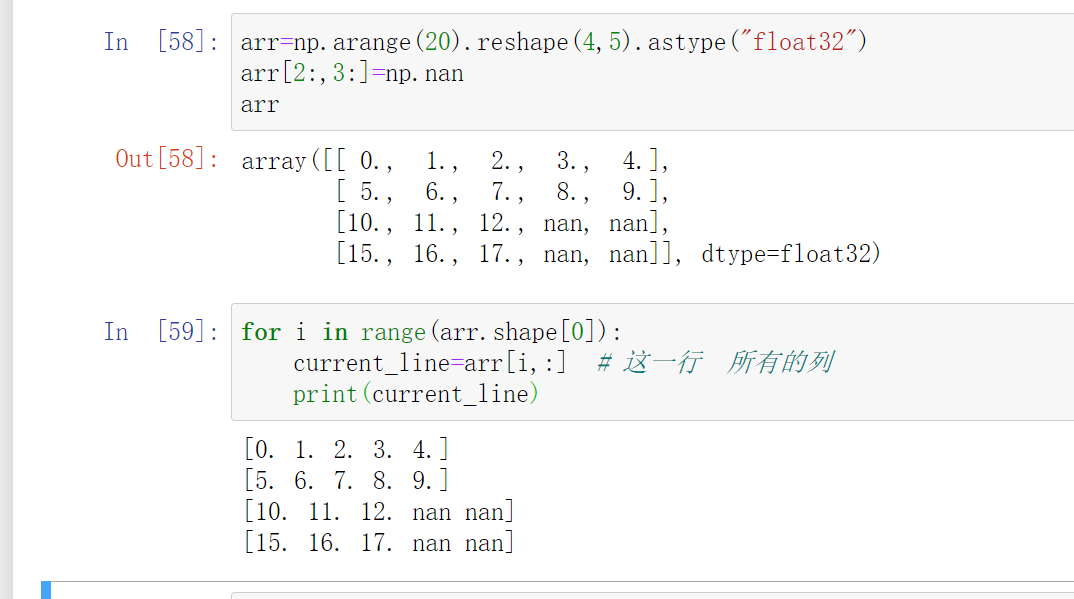### 2.如何对数组进行列遍历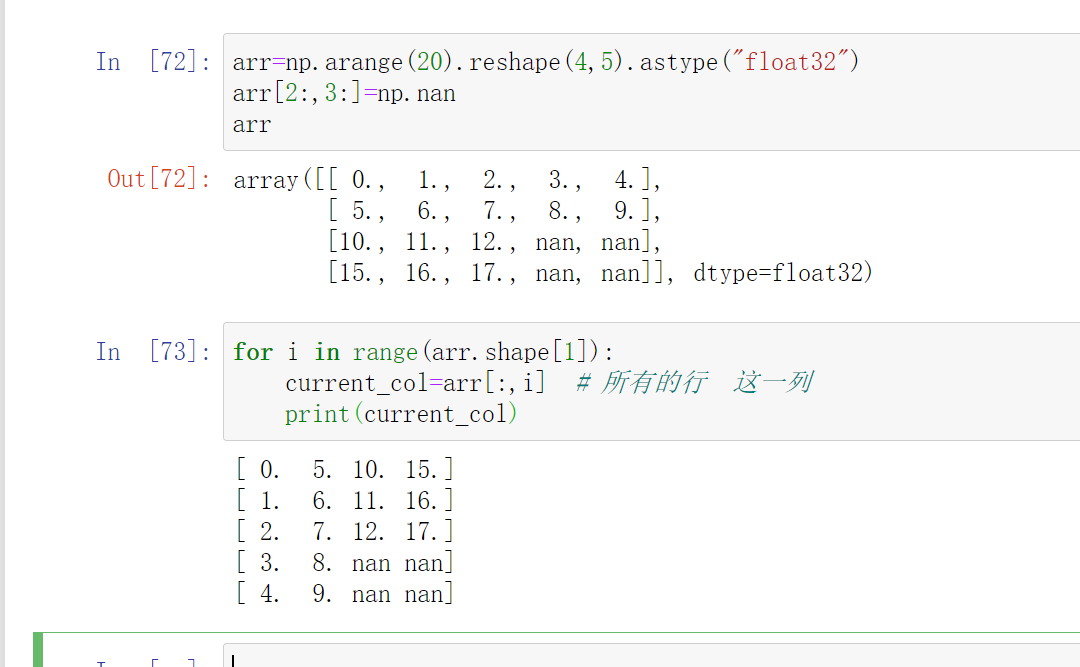### 3.检查数组中是否存在缺失值数据 如果有 有几个

#### 1.判断缺失值的数量是否为0

• `np.count_nonzero(np.isnan(arr))`
• `np.count_nonzero(arr!=arr)`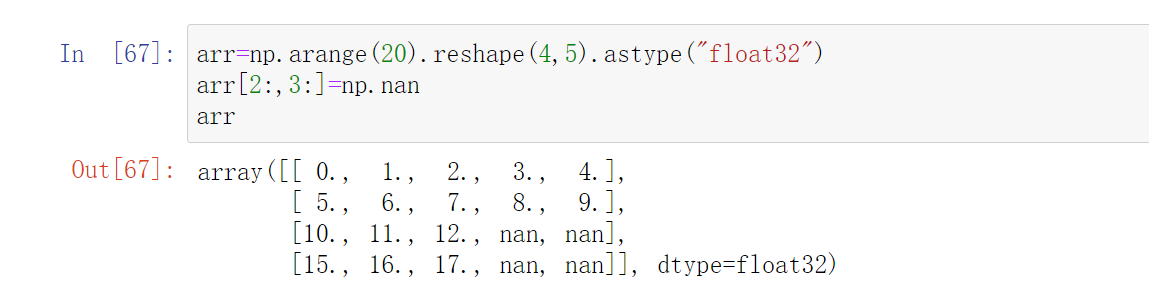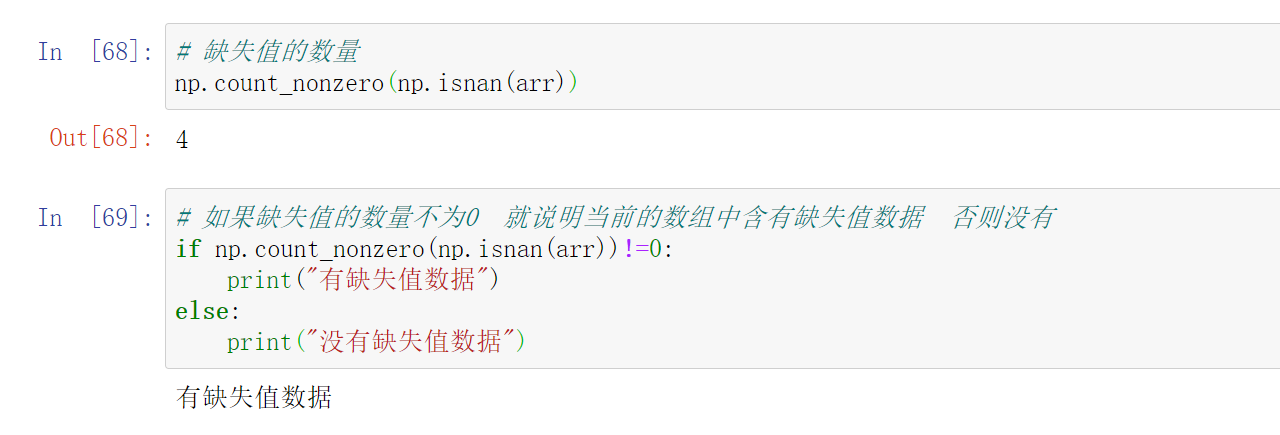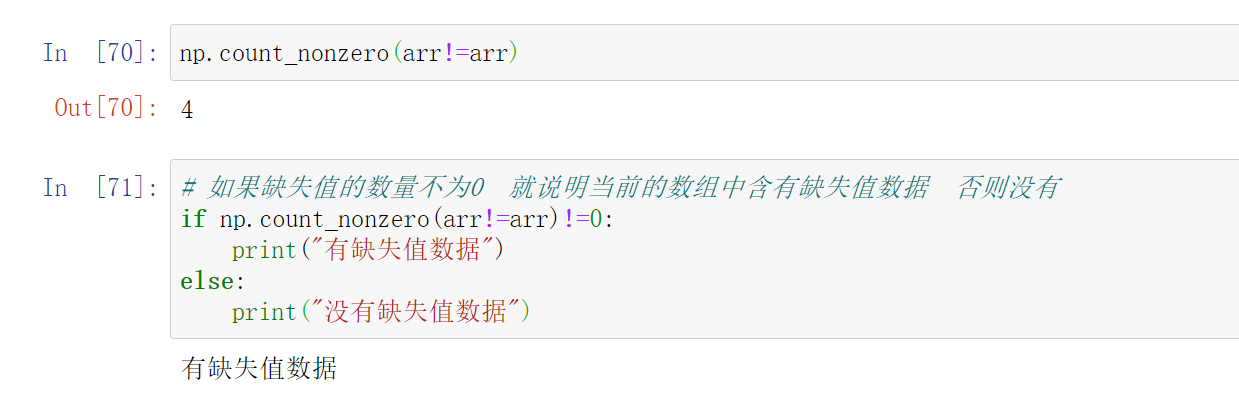#### 2.判断缺失值的数组对象是否为空

• `arr[np.isnan(arr)]`
• `arr[arr!=arr]`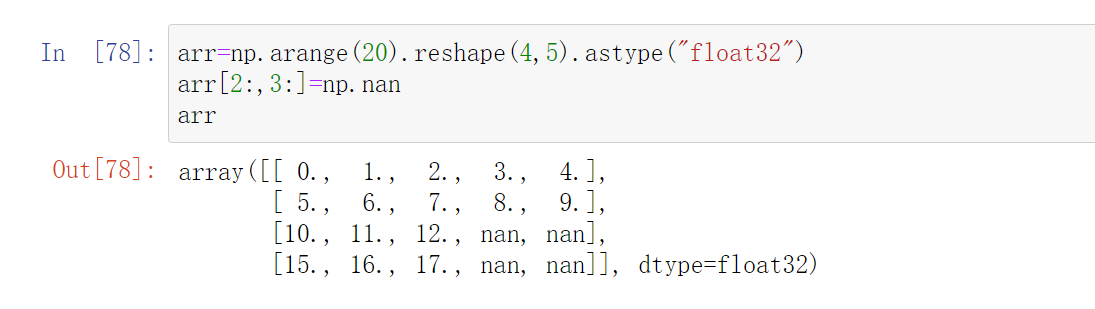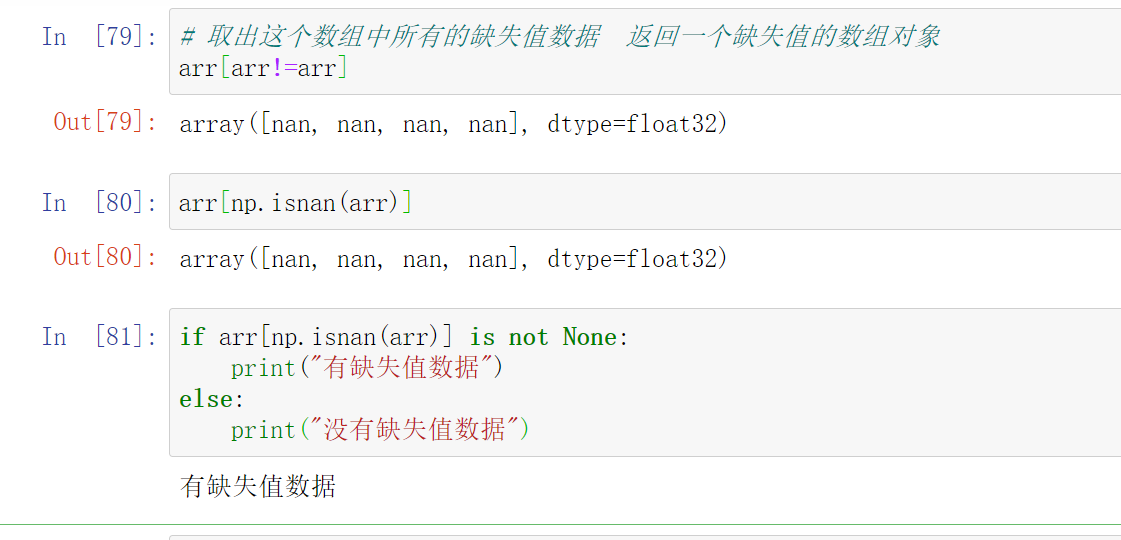• `len(arr[np.isnan(arr)])`
• `len(arr[arr!=arr])`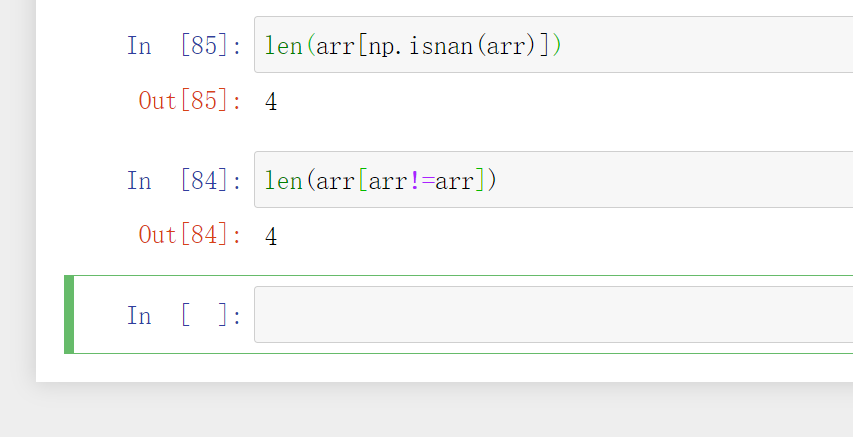## 小结

• 如何选择一行或者多行的数据（列）？
• 如何给选取的行或者列赋值？
• 如何大于把大于10的值替换为10？
• np.where如何使用？
• np.clip如何使用？
• 如何转置（交换轴）？
• 读取和保存数据为csv？
• np.nan和np.inf是什么？
• 常用的统计函数你记得几个？
• 标准差反映出数据的什么信息？

## 动手

### 例题1

#### 原始数据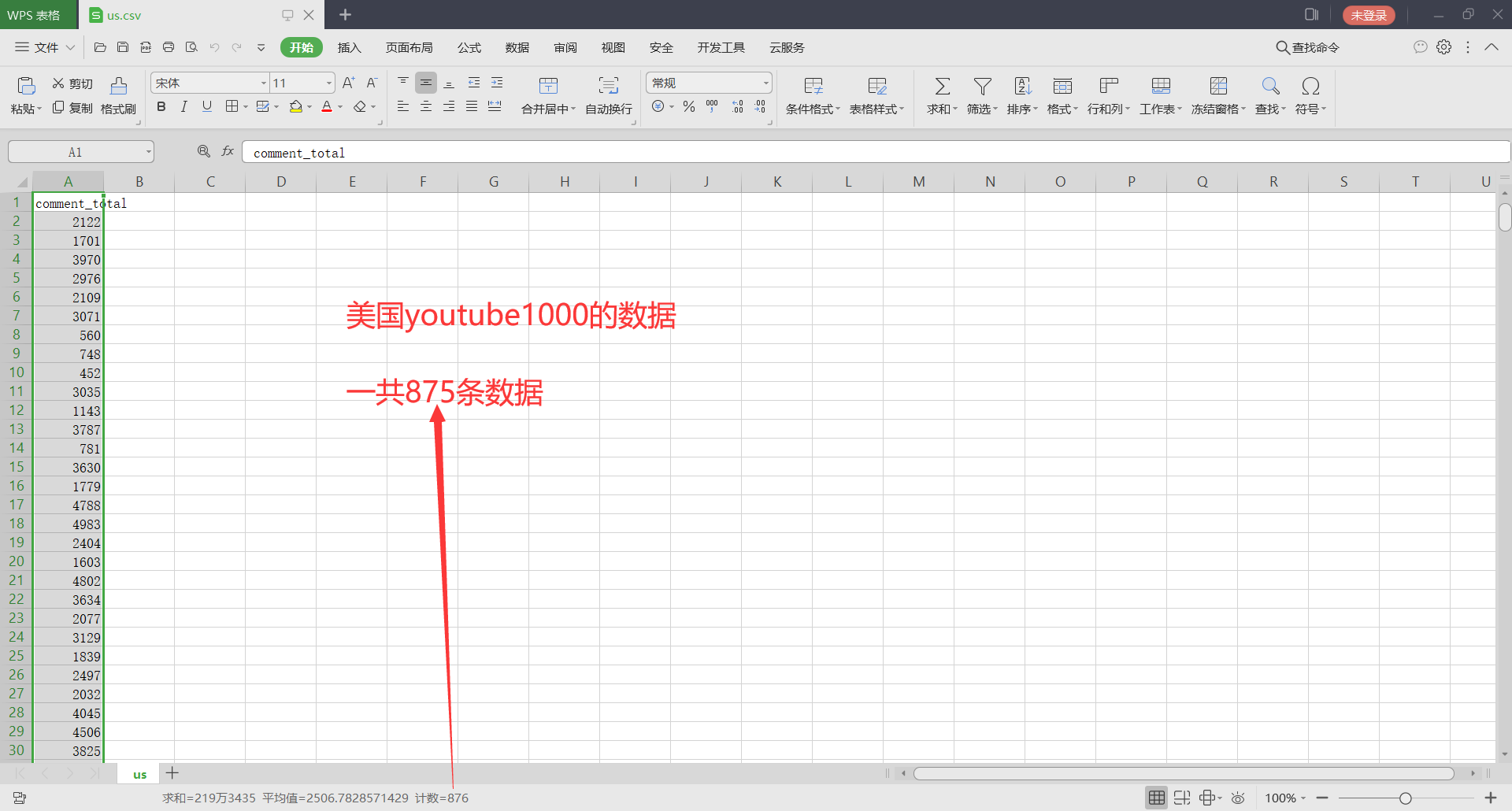#### 代码+分析+结果

``````# -*- coding: utf-8 -*-

'''
@Time    : 2020/12/08 16:24
@Author  : yuhui
@Email   : [email protected]
@FileName: numpy_3.py
@Software: PyCharm
'''

"""20【numpy中的nan和常用方法】numpy中填充nan"""

import numpy as np
import matplotlib.pyplot as plt

# 解决中文乱码
plt.rcParams["font.sans-serif"]=["KaiTi"]
plt.rcParams["font.family"]="sans-serif"
# 解决符号无法显示的问题
plt.rcParams['axes.unicode_minus'] = False # 解决保存图像时负号'-'显示为方块的问题

"""设置图形大小和图片品质"""
fig=plt.figure(
figsize=(16,9),
dpi=100,
)

us_file_path=r"D:\Python\数据分析\数据分析2\data\us.csv"

dtype="int32",  # 数据类型
delimiter=",",  # 分隔符
skiprows=1  # 跳过开头的几行
)

"""绘制直方图"""

# 打印出最大值和最小值  根据最大值和最小值确定出组距，进而确定组数

# 组距
group_spacing=250

# 组数

# 绘制直方图

color="#6495ED",  # 颜色
)

"""设置x轴刻度"""

"""绘制网格线"""
plt.grid(
linestyle=":",  # 线型
color="#6495ED",  # 颜色  CornflowerBlue	矢车菊的蓝色	#6495ED	100,149,237
alpha=0.4,  # 透明度
)

plt.show()

``````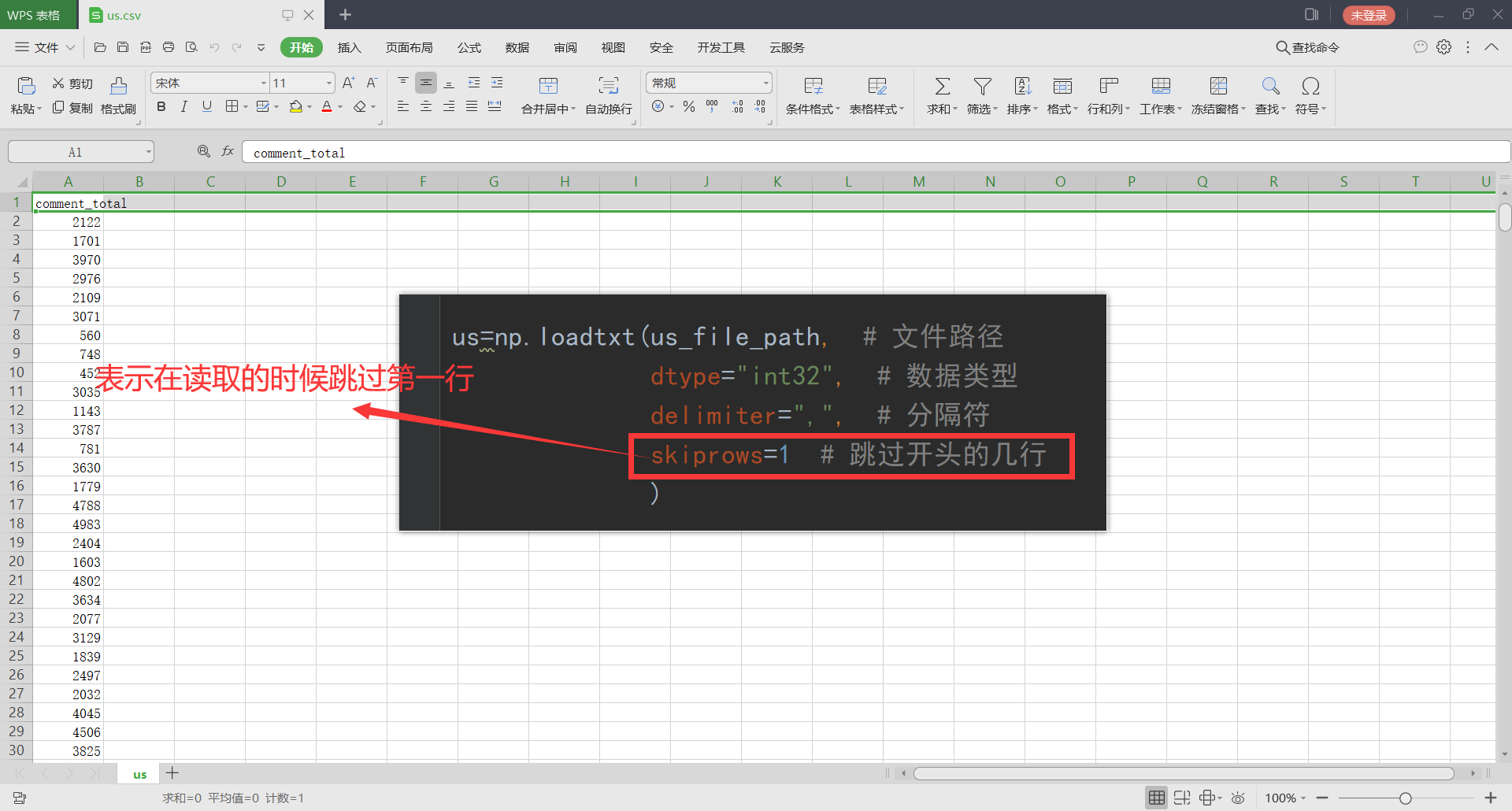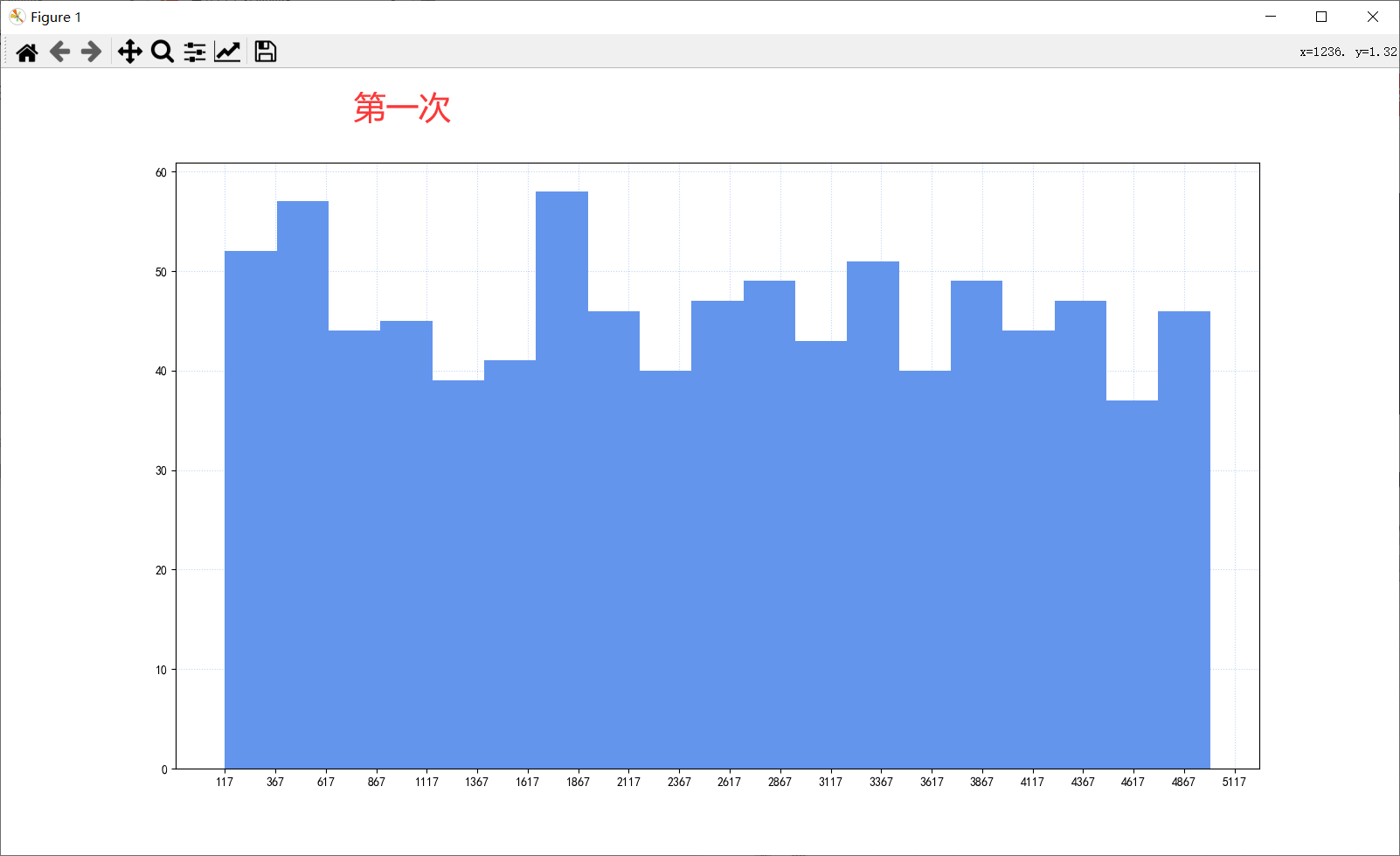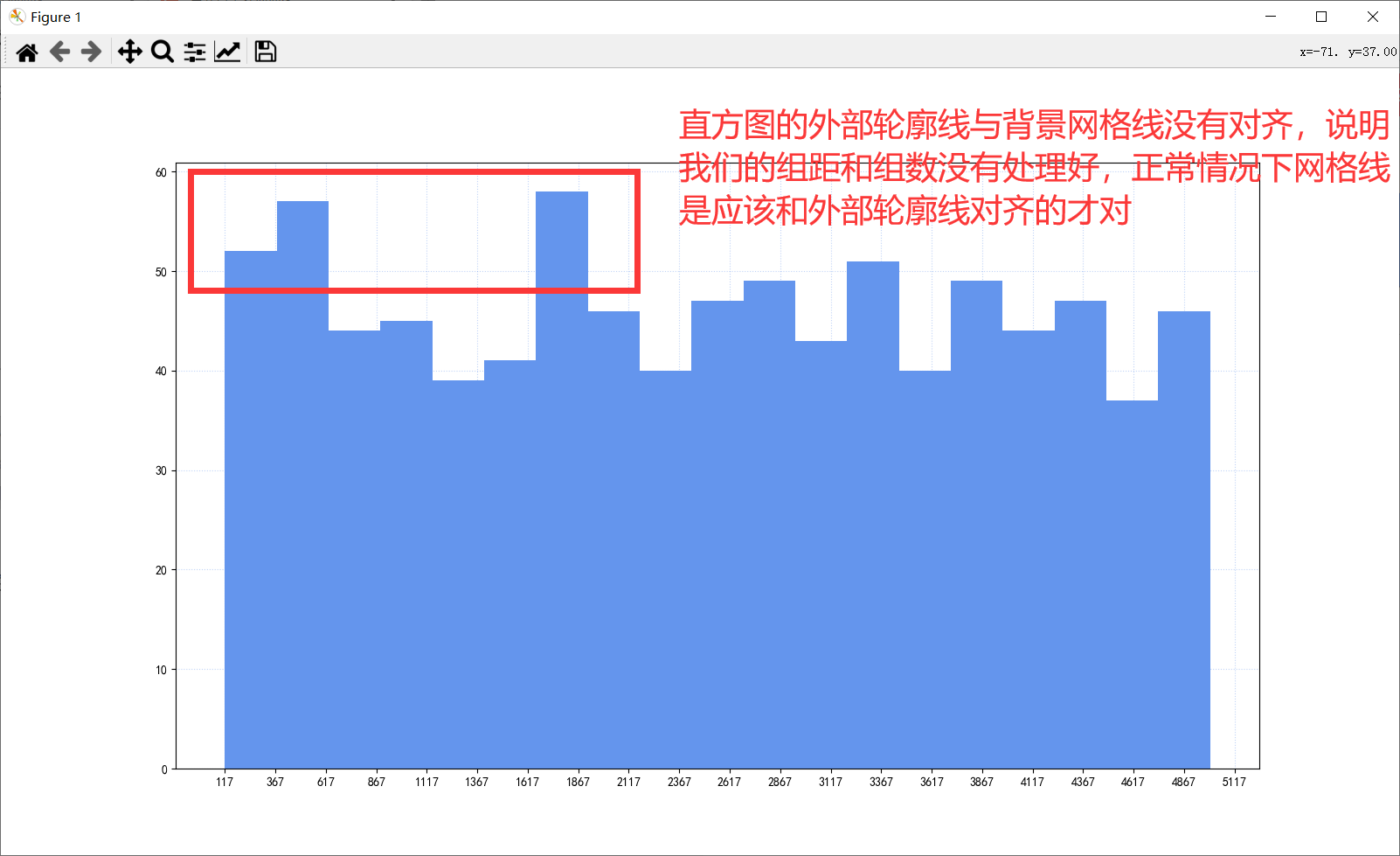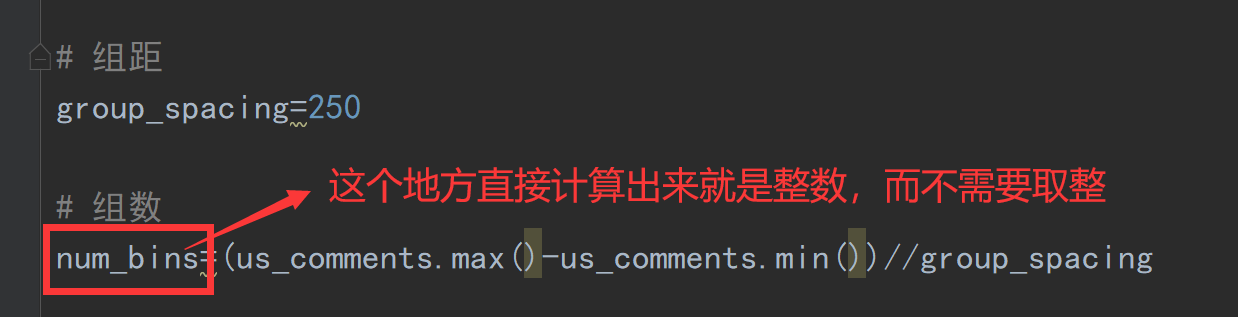``````# 打印出最大值和最小值  根据最大值和最小值确定出组距，进而确定组数

# 组距
group_spacing=17*41
# 4996-117=4879=7*17*41
# 组数
``````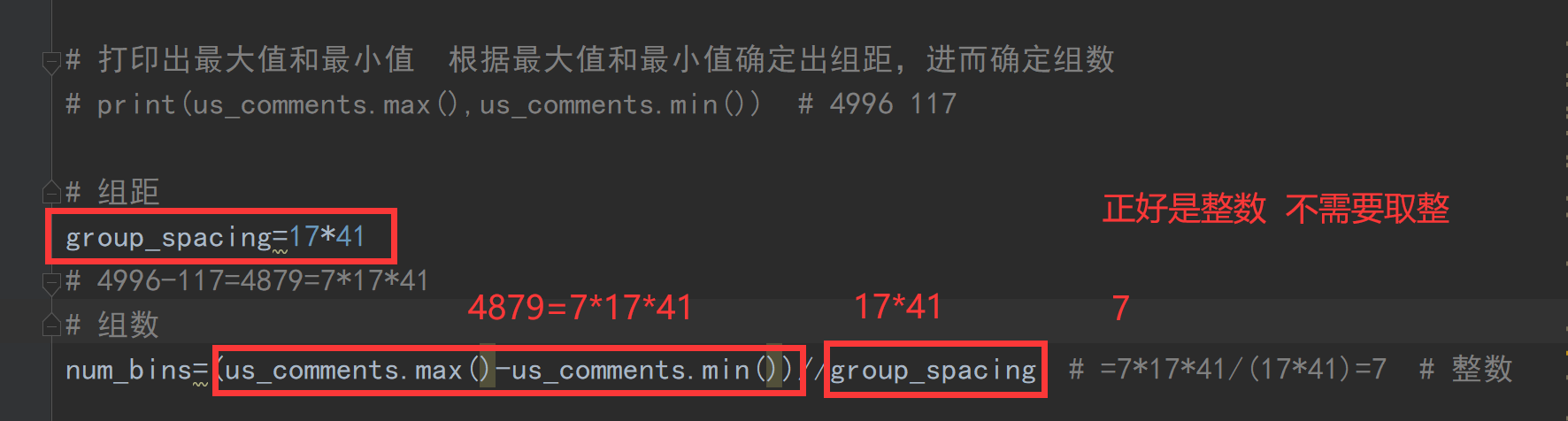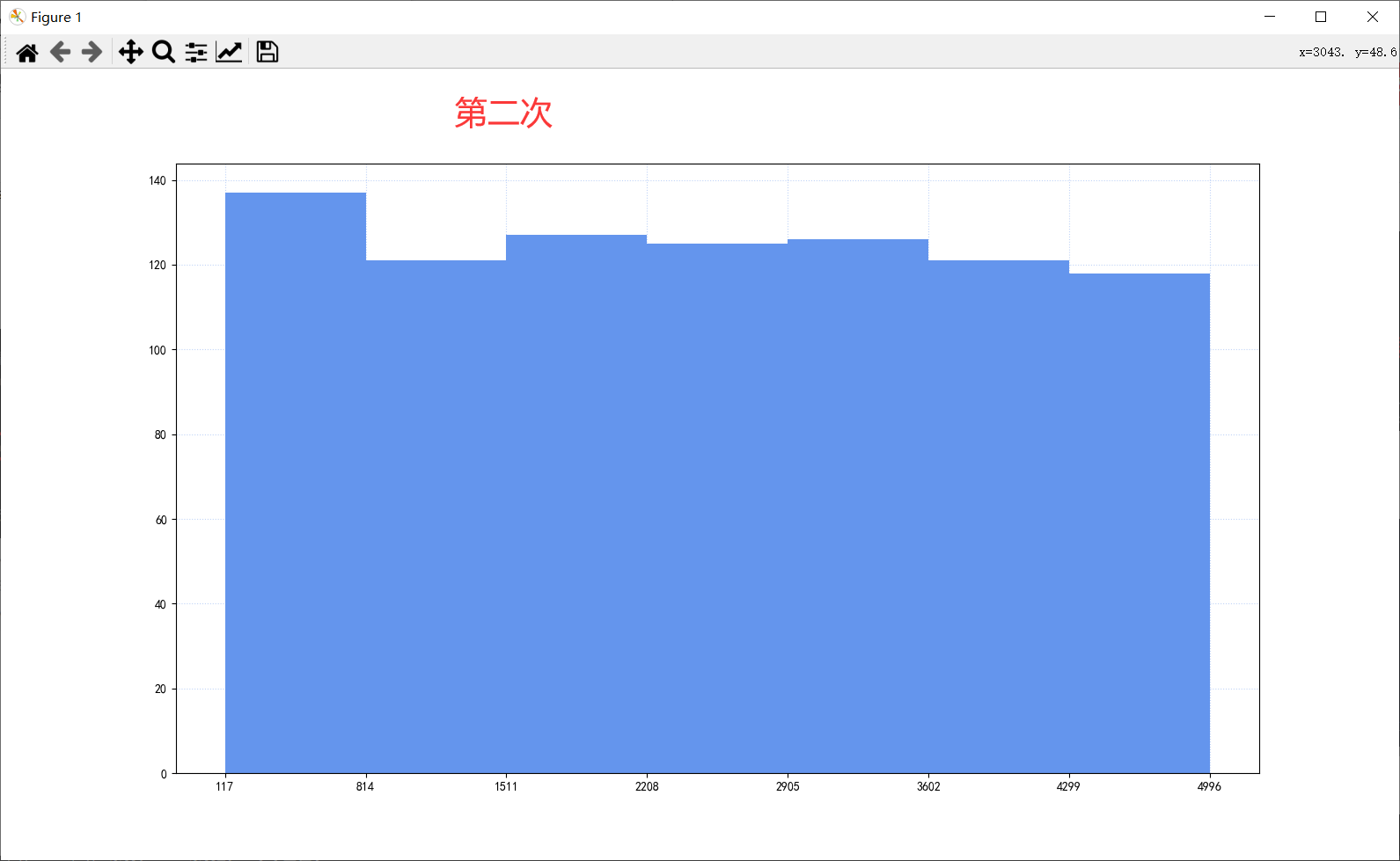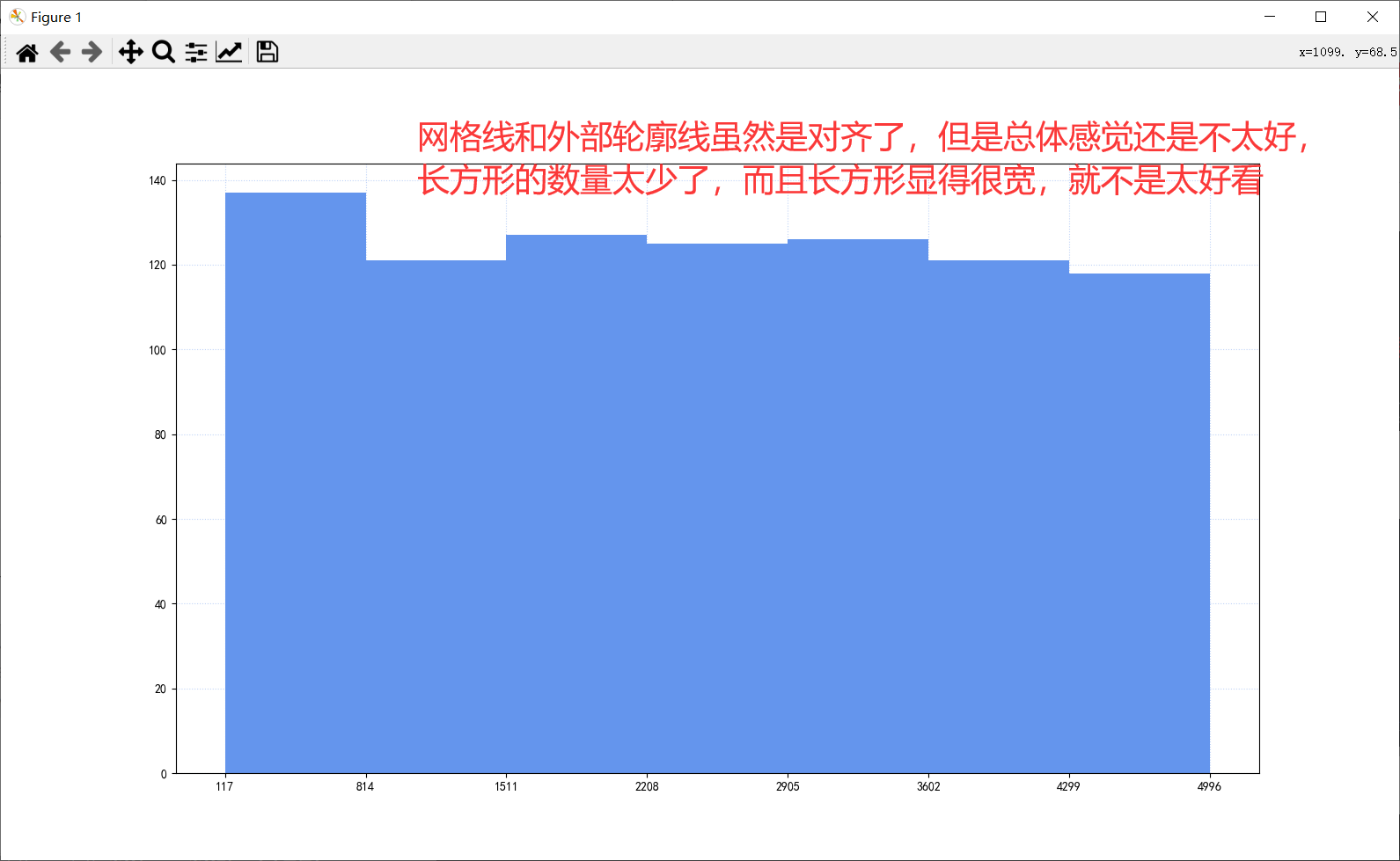``````# -*- coding: utf-8 -*-

'''
@Time    : 2020/12/08 16:24
@Author  : yuhui
@Email   : [email protected]
@FileName: numpy_3.py
@Software: PyCharm
'''

"""20【numpy中的nan和常用方法】numpy中填充nan"""

import numpy as np
import matplotlib.pyplot as plt

# 解决中文乱码
plt.rcParams["font.sans-serif"]=["KaiTi"]
plt.rcParams["font.family"]="sans-serif"
# 解决符号无法显示的问题
plt.rcParams['axes.unicode_minus'] = False # 解决保存图像时负号'-'显示为方块的问题

"""设置图形大小和图片品质"""
fig=plt.figure(
figsize=(16,9),
dpi=100,
)

us_file_path=r"D:\Python\数据分析\数据分析2\data\us.csv"

dtype="int32",  # 数据类型
delimiter=",",  # 分隔符
skiprows=1  # 跳过开头的几行
)

"""绘制直方图"""

# 打印出最大值和最小值  根据最大值和最小值确定出组距，进而确定组数

# 组距
group_spacing=17*41
# 4996-117=4879=7*17*41
# 组数

# 绘制直方图
color="#6495ED",  # 颜色
)

"""设置x轴刻度"""

"""绘制网格线"""
plt.grid(
linestyle=":",  # 线型
color="#6495ED",  # 颜色  CornflowerBlue	矢车菊的蓝色	#6495ED	100,149,237
alpha=0.4,  # 透明度
)

plt.show()

``````

### 例题2

#### 原始数据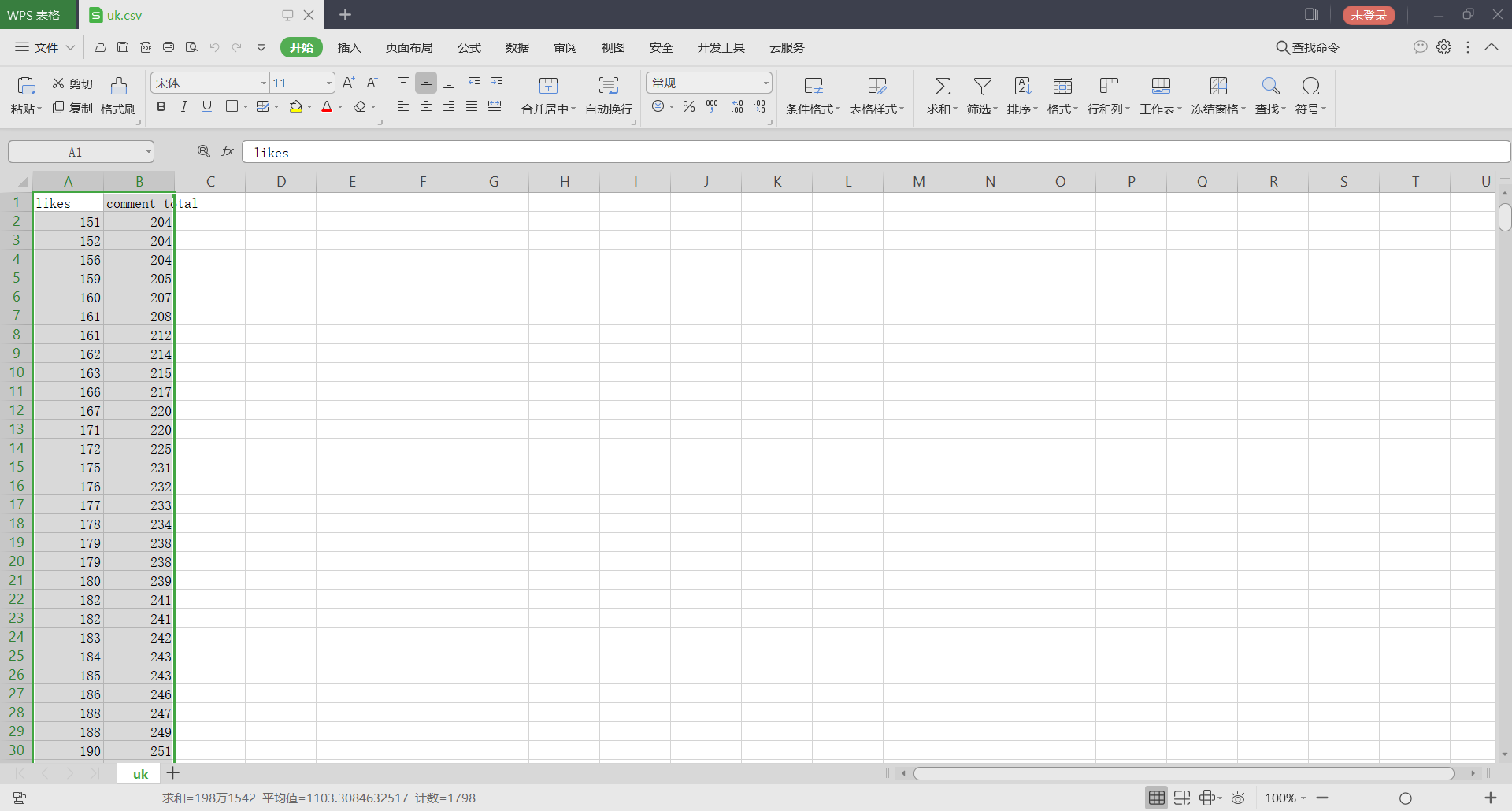#### 代码+分析+结果

``````# -*- coding: utf-8 -*-

'''
@Time    : 2020/12/08 16:36
@Author  : yuhui
@Email   : [email protected]
@FileName: numpy_4.py
@Software: PyCharm
'''

"""20【numpy中的nan和常用方法】numpy中填充nan"""

import numpy as np
import matplotlib.pyplot as plt

# 解决中文乱码
plt.rcParams["font.sans-serif"]=["KaiTi"]
plt.rcParams["font.family"]="sans-serif"
# 解决符号无法显示的问题
plt.rcParams['axes.unicode_minus'] = False # 解决保存图像时负号'-'显示为方块的问题

"""设置图形大小和图片品质"""
fig=plt.figure(
figsize=(16,9),
dpi=100,
)

uk_file_path=r"D:\Python\数据分析\数据分析2\data\uk.csv"

dtype="int32",
delimiter=",",
skiprows=1,  # 跳过第一行
)

likes=uk[:,0]  # 纵轴数据  第一列

"""绘制散点图"""

plt.show()

``````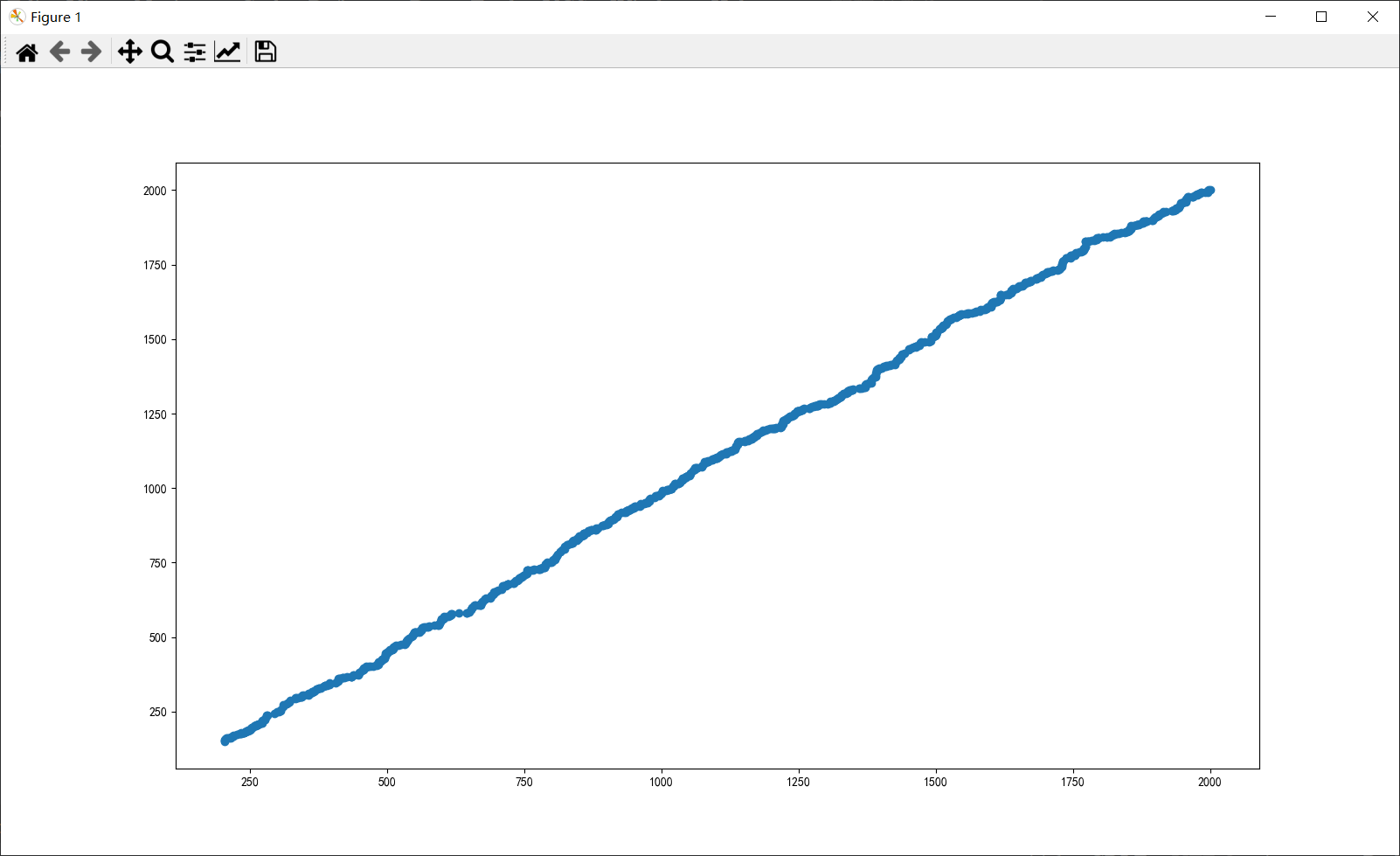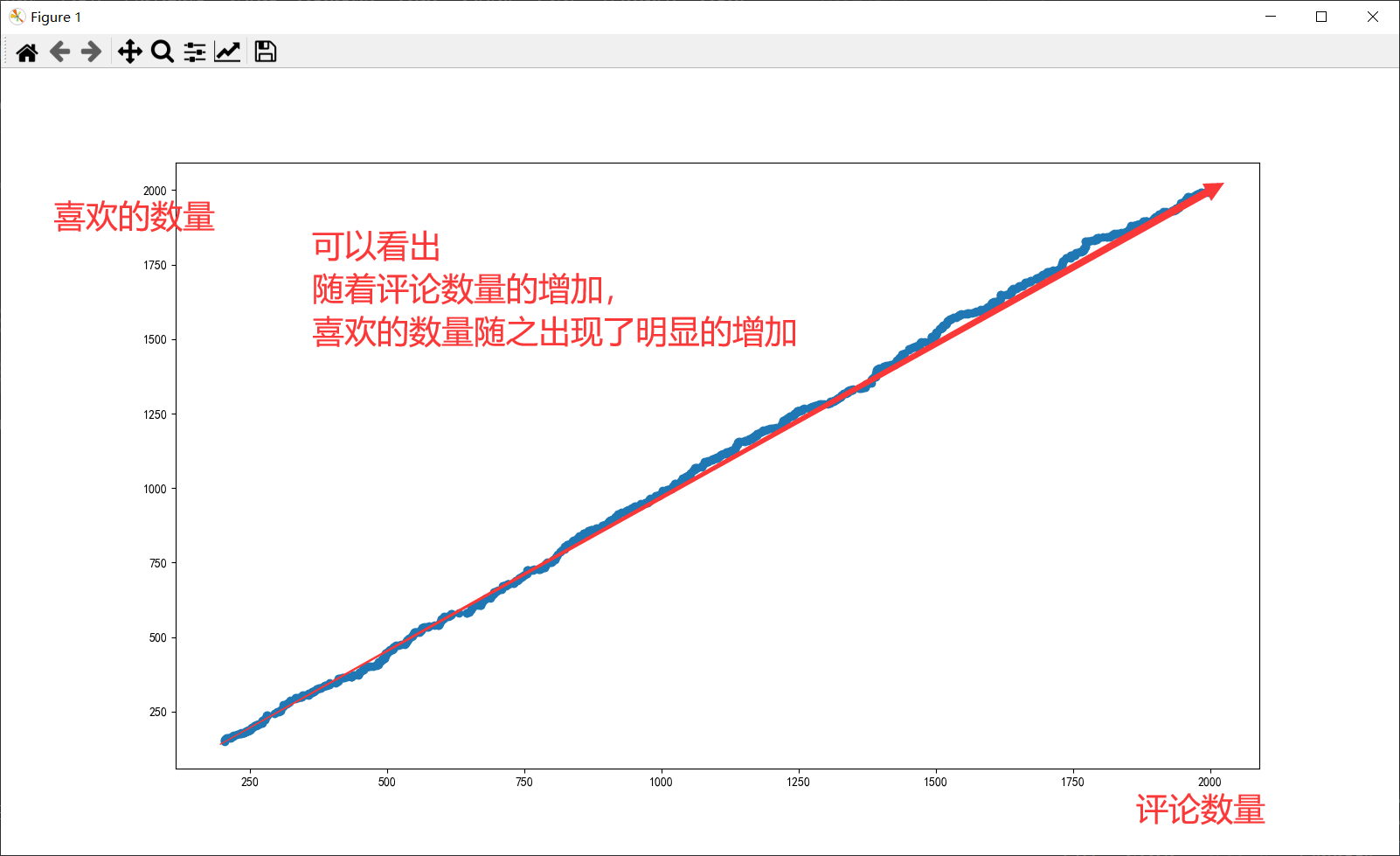### psid mysql_DB2常用SQL的写法(持续更新中...)_栗迦南的博客-程序员秘密

DB2常用SQL的写法(持续更新中...)-- Author: lavasoft-- Date : 2006-12-14-- 创建一个自定义单值类型create distinct type var_newtypeas decimal(5,2) with comparisons;-- var_newtype 类型名-- decimal(5,2) 实际的类型-- 删除一个自定义单值类型drop ...

### python读取目录下所有的jpg文件，并显示第一张图片_文件夹显示第一张_xky1306102chenhong的博客-程序员秘密

# -*- coding: UTF-8 -*-import numpy as npimport osfrom scipy.misc import imread, imresizeimport matplotlib.pyplot as pltfrom glob import glob# 读取目录下所有的jpg图片def load_image(image_path, image_s...

### 阿里云天池Python训练营（day5打卡）_陈ty0712的博客-程序员秘密

【ML&amp;Py】×阿里云天池Python训练营（day4打卡）——1.字符串、2.字典一、学习概览1.1 学习地址：[阿里云天池python训练营](https://tianchi.aliyun.com/specials/promotion/aicamppython)1.2 思维导图二、具体学习内容2.1 字符串2.1.1 字符串的定义2.1.2 字符串的切片与拼接2.1.3 字符串的常用内置方法2.1.4 字符串格式化2.2 字典2.2.1 可变类型与不可变类型2.2.2 字典的定义2.2.3 创建